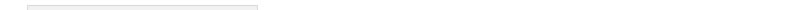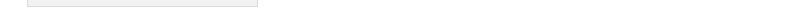Sitemap Print Version Mailform Login Last update:April 15. 2010 14:22:22

Math 579 > Matlab files

# Matlab files

Here you can find some m-files with commentaries. To see the commentary, type

>> help filename

in Matlab command window. (here 'filename' should be replaced by actual name, for instance, euler).

Disclaimer: These files are provided "as is", without warranties of any kind.

Some fixed-stepsize Runge-Kutta type solvers for initial value problems:
• Euler's method for scalar equations: euler1.m
• Heun's method for scalar equations: heun1.m
• The midpoint method for scalar equations: midpoint1.m
• (General) Euler's method: euler.m
• (General) Heun's method: heun.m
• The (general) midpoint method: midpoint.m
• Runge-Kutta method of order 4: rk4.m
One step at a time:
Stability region:
Some examples of modeling and simulation by IVPs:
Variable step-size (aka adaptive) methods:
• Runge-Kutta-Fehlberg order 4/order 5 embedded pair: rkf45.m
Implicit methods:
• Backward Euler with Newton's method as a solver (fixed step-size): beuler.m
Equation solvers:
• A simple implementation of Newton's method: newton.m
• A simple implementation of the secant method: secant.m
Multistep methods:
• Adams-Bashforth 4-step method: ab4.m
• Adams-Bashforth / Adams-Moulton predictor-corrector pair of order 4: abm4.m
Shooting methods for 2nd order (Dirichlet) boundary value problems:
• Linear shooting method (with fixed stepsize IVP solvers): linshoot.m
• Shooting method using bisection (with fixed stepsize IVP solvers): bisectshoot.m
• Shooting method using bisection (with Runge-Kutta-Fehlberg 4/5 variable stepsize solver): rkf45bisectshoot.m
Finite difference methods for 2nd order (Dirichlet) boundary value problems:
• For linear problems: linfd.m
• For nonlinear problems (using a fixed point iteration): fpifd.m
• For nonlinear problems (using Newton's iteration): newtfd.mMATH 579: Numerical Differential Equations Winter 2010